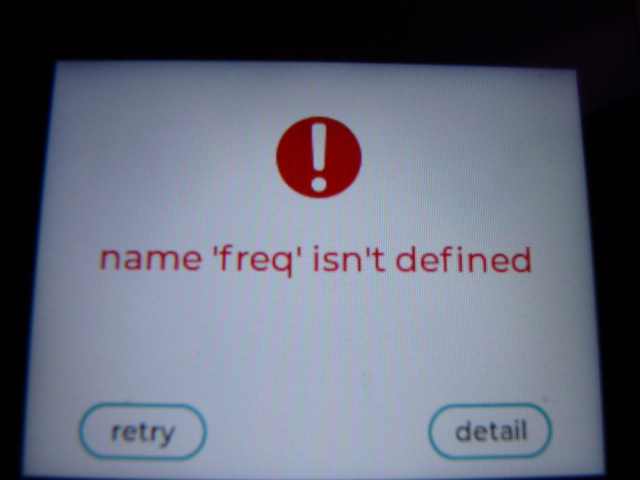# Not possible to connect Pa.HUB on M5Stack CORE2

• Hello together
I have a problem to connect the Pa.HUB to my CORE2

I try to do a weatherstation, were I have to read from different sensor's
I have to use "ENVII", "EXT.I/O" and "ADC" sensors. I try to use the Pa.HUB as connector.
The Pa.HUB is connectet to the PortA (Red)
Always when I try to uploade the programm, there is the fail: "name 'freq' isn't defined"

When I connect the ENVII directly to PortA, since V1.7.2 it works. But i need to read more sensors.

I use the M5 CORE2, with PaHUB
I use the V1.7.2 on my M5 and did burn them with M5Burner.
I use the V1.7.2 UiFLOW Beta (The other version do not support the M5 Core2)
The sending/uploding from the progrogrammcode to the M5 over the Internet work's well with other codes, the country is switzerland.

codeexample:
~~
from m5stack import *
from m5stack_ui import *
from uiflow import *
import time
import unit

screen = M5Screen()
screen.clean_screen()
screen.set_screen_bg_color(0xFFFFFF)
pahub1 = unit.get(unit.PAHUB, unit.PORTA)
env21 = unit.get(unit.ENV2, unit.PAHUB0)
ext_io1 = unit.get(unit.EXT_IO, unit.PAHUB1)

text_header = M5Label('Wetterstation Transferprojekt', x=15, y=10, color=0x000, font=FONT_MONT_26, parent=None)
text2_humi = M5Label('Luftfeuchtigkeit:', x=15, y=75, color=0x000, font=FONT_MONT_18, parent=None)
text1_temp = M5Label('Temparatur:', x=15, y=50, color=0x000, font=FONT_MONT_18, parent=None)
text3_pres = M5Label('Luftdruck:', x=15, y=100, color=0x000, font=FONT_MONT_18, parent=None)
text3_windspeed = M5Label('Windgeschwindigkeit:', x=15, y=125, color=0x000, font=FONT_MONT_18, parent=None)
text5_winddir = M5Label('Windrichtung:', x=15, y=150, color=0x000, font=FONT_MONT_18, parent=None)
text6_rain = M5Label('Regen:', x=15, y=175, color=0x000, font=FONT_MONT_18, parent=None)
val_temp = M5Label('N/A', x=200, y=50, color=0x000, font=FONT_MONT_18, parent=None)
val_humi = M5Label('N/A', x=200, y=75, color=0x000, font=FONT_MONT_18, parent=None)
val_pres = M5Label('N/A', x=200, y=100, color=0x000, font=FONT_MONT_18, parent=None)
val_windspeed = M5Label('N/A', x=200, y=125, color=0x000, font=FONT_MONT_18, parent=None)
val_winddir = M5Label('N/A', x=200, y=150, color=0x000, font=FONT_MONT_18, parent=None)
val_rain = M5Label('N/A', x=200, y=175, color=0x000, font=FONT_MONT_18, parent=None)

while True:
val_temp.set_text(str(env21.temperature))
val_humi.set_text(str(env21.humidity))
val_pres.set_text(str(env21.pressure))
wait(2)
wait_ms(2)~~

Error Detail
~~
File "<string>", line 11, in <module>
File "unit.py", line 130, in get
File "<string>", line 1, in <module>
File "<units/_env2.py>", line 7, in init
File "<i2c_bus.py", line 183, in init
File "<i2c_bus.py", line 40, in init
File "<i2c_bus.py", line 32, in get
File "<i2c_bus.py", line 130, in init
NameError: name 'freq' isn't defined
~~

Hope for a solution
Thx Matthias

• I have exactly the same error message with my CORE2 connected to a PaHub and an ADC module.
The ADC module works perfectly directly connected to port A of CORE2, but not through the PaHub• The error should not be connected to where the device is conneced.
`NameError: name 'freq' isn't defined`
`Device not found, check connection`

I see That you are using the Core2 so its possible that 'freq' is called something else and you have found another bug in UIFlow

• I have the same problem with the PaHub and two ToF sensors.
Firmware Used: `v1.7.2-core2`

Simple code to reproduce below. And Screenshot for graphical representation.

``````from m5stack import *
from m5stack_ui import *
from uiflow import *
import unit

screen = M5Screen()
screen.clean_screen()
screen.set_screen_bg_color(0xFFFFFF)
pahub0 = unit.get(unit.PAHUB, unit.PORTA)
tof0 = unit.get(unit.TOF, unit.PAHUB0)
tof1 = unit.get(unit.TOF, unit.PAHUB1)

label0 = M5Label('Text', x=21, y=13, color=0x000, font=FONT_MONT_46, parent=None)
label1 = M5Label('Text', x=21, y=71, color=0x000, font=FONT_MONT_46, parent=None)

while True:
label0.set_text(str(tof0.distance))
label1.set_text(str(tof1.distance))
wait_ms(2)
``````• Hi guys

not ideal at all but here is a possible work-a-round until this stupid issue has been resolved.

The trick is to not add the units connected to PaHUB in UiFlow but to initialise them later when PaHUB has selected the respective channel / unit.
In my example the AMeter is connected to PaHUB output 0. Also variable `current` needs to be defined from within UiFlow.``````from m5stack import *
from m5stack_ui import *
from uiflow import *
import unit

screen = M5Screen()
screen.clean_screen()
screen.set_screen_bg_color(0xFFFFFF)
pahub0 = unit.get(unit.PAHUB, unit.PORTA)

current = None

label0 = M5Label('Text', x=30, y=43, color=0x000, font=FONT_MONT_24, parent=None)

pahub0.select_only_on(0)
myAMeter = unit.get(unit.AMETER, (32, 33))
current = str(myAMeter.current())
label0.set_text(str(current))
``````

Thanks
Felix

• Hello again

I found that when I switch M5Core2 firmware back to 1.7.1.1 and use it with UIFlow 1.7.2 (online) the error goes away and PaHUB works as expected.

Thanks
Felix